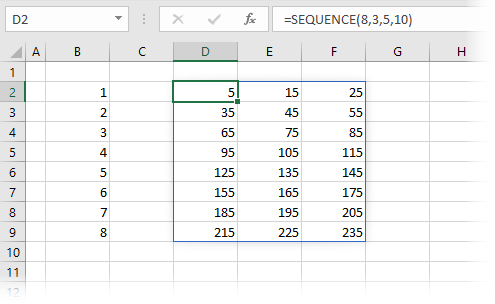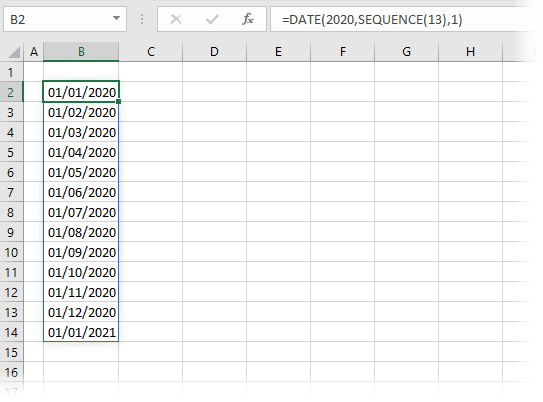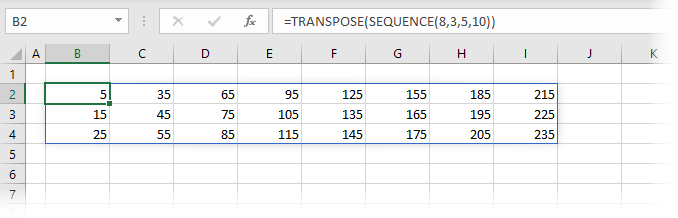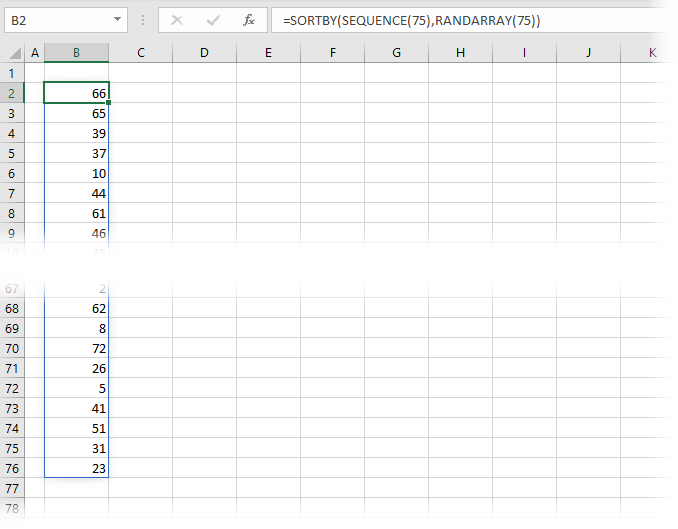Get our FREE VBA eBook of the 30 most useful Excel VBA macros.

Automate Excel so that you can save time and stop doing the jobs a trained monkey could do.

# SEQUENCE function in ExcelThe SEQUENCE function is one of the new dynamic array functions which Microsoft released as part of introducing dynamic arrays. This function makes use of changes to Excel’s calculation engine, which enables a single formula to display (or “spill” if using the new terminology) results in multiple cells. In the case of SEQUENCE, it will generate a list of sequential numbers in a two-dimensional array across both rows and columns.

At the time of writing, the SEQUENCE function is only available to those on a Microsoft 365 subscription. It will not be available in Excel 2019 or earlier versions.

I recommend you download the example file for this post. Then you’ll be able to work along with examples and see the solution in action, plus the file will be useful for future reference.Watch the video:

Contents

## The arguments of the SEQUENCE function

The SEQUENCE function has four arguments; Rows, Columns, Start, Step.

```=SEQUENCE(Rows, [Columns], [Start], [Step])
```
• Rows: The number of rows to return
• [Columns] (optional): The number of columns to return. If excluded, it will return a single column.
• [Start] (optional): The first number in the sequence. If excluded, it will start at 1.
• [Step] (optional): The amount to increment each subsequent value. If excluded, each increment will be 1.

Please note, while Excel creates sequences in rows and columns, it moves across columns, before moving down to the next row. Look at Example 3 to learn how to flip that functionality around.## Examples of using the SEQUENCE function

The following examples illustrate how to use the SEQUENCE function. As a dynamic array function, the result will automatically spill into neighboring rows and columns.

### Example 1 – Basic Usage

The two examples below show the basic usage of the SEQUENCE function to provide a sequence of numbers.

Excluding optional argumentsThe formula in cell B2 is:

```=SEQUENCE(8)
```

In this formula, only the Rows argument is provided; all the other arguments ([Columns] optional, [Start] optional, and [Step] optional), have been excluded. Therefore, the default values are applied for each of these. SEQUENCE has created a list of sequential numbers; 8 rows, 1 column, starting at 1 and incrementing by 1 for each cell.

Including all argumentsThe formula in cell D2 uses all the arguments: Rows, Columns, Start, Step.

```=SEQUENCE(8,3,5,10)
```

When using the rows and columns arguments it creates sequential numbers in a two-dimensional array. The formula is creating an array with:

• Rows argument = 8
• Columns argument = 3
• Start argument = 5
• Step argument = 10

The order of the numbers is important here, the function increments across the columns before moving to the next row.

### Example 2 – Using SEQUENCE inside other functions

The example below shows how to use SEQUENCE inside the DATE function.

Note: As I am based in the UK, the screenshot below shows the UK date format (dd/mm/yyyy).The formula in cell B2 is:

```=DATE(2020,SEQUENCE(13),1)
```

This formula creates a sequence of monthly dates starting on January 1st, 2020. The SEQUENCE function has been applied to the month argument; therefore, it adds one to the month for each subsequent result. We have requested 13 months, but Excel is intelligent enough to know that 01/13/2020 doesn’t exist as a UK date format. Instead, the DATE function will cause the result to roll-over into the next year and create 01/01/2021.

### Example 3 – SEQUENCE row / column order

As already mentioned, the SEQUENCE formula in Excel creates sequential numbers across both rows and columns. However, please note that by default they increment across columns before going down to the next row. To change this, we can use the TRANSPOSE function.The following formula in cell B2 is:

```=TRANSPOSE(SEQUENCE(8,3,5,10))
```

This is the same SEQUENCE function as we used in Example 1, but it has been wrapped in TRANSPOSE. Note that now the numbers increment down each row before going to the next column.

This can get a little confusing as while we have used the rows and columns arguments, the rows argument is displaying 8 columns and the column argument is displaying 3 rows. When we use this technique, we just need to remember that TRANSPOSE is applied as the last action.

### Example 4 – Sequence with INDEX

The SEQUENCE formula in Excel is useful by itself, but when combined with other functions it’s power can be clearly seen.

In the screenshot below, we have a list of names and then the person’s ID or department. There are two rows for every individual. How can we get a list of just the ID’s sorted by last name? Let’s find out:The formula in cell F2 is:

```=SORT(INDEX(B3:D12,SEQUENCE(COUNTA(B3:B12)/2,1,1,2),SEQUENCE(1,3)),2)
```

Let’s break this down:

• SEQUENCE(COUNTA(B3:B12)/2,1,1,2) – this part uses COUNTA to calculate the number of rows, then divides that by 2, as we want to return every 2nd row. SEQUENCE is then used to create the list which is 1 column, starts at the first row but steps by 2.
• SEQUENCE(1,3) – as we have 3 columns in our source, we want to return the same 3 columns. This creates the array list for INDEX to use.
• INDEX(B3:D12,…..) – The first SEQUENCE function is returning the value {1, 3, 5, 7, 9, 11}, the second SEQUENCE function is returning the values {1;2;3}. INDEX returns only the values from B3-B12 which are in these row and column positions.
• SORT(….,2) – finally the result is sorted by the 2nd column, which is the last name

This demonstrates the power of SEQUENCE as an enabler to more complex functions.

### Example 5 – SEQUENCE in random order

Do you play bingo? No, me neither. But it creates an interesting example. I’m led to believe there are 75 numbers and those numbers can only appear once. By combining SORTBY, RANDARRAY and SEQUENCE we can put the sequence of numbers into a random order (just like bingo).The formula in cell B2 is:

```=SORTBY(SEQUENCE(75),RANDARRAY(75))
```

The SEQUENCE function returns a list from 1 to 75. This list is sorted by another list of 75 random numbers created by RANDARRAY.

### Other examples

My post about the SORT function also contains an example of using the SEQUENCE formula in Excel to create a list of the top 5 results.

There is a lot to learn about dynamic arrays and the new functions. Check out my other posts here to learn more:Get our FREE VBA eBook of the 30 most useful Excel VBA macros.

Automate Excel so that you can save time and stop doing the jobs a trained monkey could do.

Don’t forget: